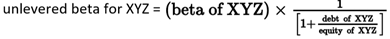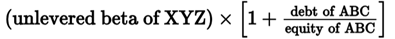# Module 27.1 – Equity Pricing Models (Ibbotsen-Chen, CAPM, Fama-French), Equity Risk Premiums & Beta Adjustments

Investors generally require a risk-premium in excess of the risk-free rate to justify holding equity securities. The risk premium in can be described as the difference between the required return on an equity index and the risk-free rate.

#### CAPM

This equation relates to the most commonly used risk-premium estimator called the CAPM model. The risk-free rate should correspond with the time horizon when using the CAPM model.

• CAPM: required rate of return = RF + β[E(RM)−RF]

Besides the CAPM model, there are other models which we can use to estimate the risk-free rate based on the inputs we are given. For these, the risk-free rate generally refers to a short-term T-bill.

#### Multifactor Models

Multifactor models could be understood as an expansion on the CAPM model, which is a single factor model. In a multifactor model, the required return is the sum of the risk-free rate plus n-factor risk premiums. Each risk premium is equal to a factor sensitivity or beta multiplied by its factor risk premium.

• real required rate of return = country return +/- industry adjustment =/- size adjusment =/- leverage adjustment

#### Fama-French Model

The Fama-French model is a multi-factor model designed for small-cap required rate of return estimations.

The require rate of return = rf + (Sensitivity to equity market factor × Equity risk premium) + (Sensitivity to size factor × Size risk premium) + (Sensitivity to value factor × Value risk premium)

or

• i = rF + βmktiRMRF + βsizeiSMB + βvalueiHML

#### Gordon Growth Model

Also known as the Constant Growth model, this method calculates the risk premium from an equation derived from the Gordon Growth Model.

Risk premium = (D­1/P) + g­ – r­f,LT

The GGM risk premium is the equal to the dividend yield on a market index plus a consensus long-term earnings growth rate, minus the long-term government bond yield.

This model’s weaknesses lies in the fact that dividends are subject to boom-bust period adjustments, and the need for a stable dividend assumption.

#### Ibbotson Chen Model

Equity risk premium = {[(1+EINFL)(1+EGREPS)(1+EGPE) − 1.0] + EINC} – Expected risk-free return

The model is straightforward enough, the key is to remember the equation and the inputs.

EINFL is expected inflation, EGREPS is expected earnings growth, EGPE is expected change in the PE ratio, and EINC is expected index yield.

We can derive EGPE as the sum of the labor productivity growth rate and the labor supply growth rate, in other words, EGPE can be approximated as the real GDP growth rate.

#### Macroeconomic Multifactor Models

These are factor models that use economic variables that are likely related to cash flows and discount rates. In the Burmeister, Roll and Ross model the CFA uses, the 5 following factors are presented:

1. Confidence risk – unexpected change between return of risky corporate bonds and government bonds
2. Time horizon risk – unexpected change between the return of long-term government bonds and T-bill
3. Inflation risk – unexpected change in inflation rate
4. Business cycle risk – unexpected change in the level of real business activity
5. Market timing risk – equity market return not explained by other 4 factors

To calculate the required return on equity, multiply each factor value by a beta and add the products to the risk free rate.

#### Build-up Method

The build up method is most used on closely-held companies that do not have readily available betas. The required return is simply the sum of the risk free rate and several risk premium, for instance:

#### Bond-Yield Plus Risk Premium Method

This method is the same as the build-up method but the incorporates publicly traded debt of  the firm. We simply add a risk premium to the long-term YTM of the company’s debt.

#### Adjusted Beta for Public Companies

Some analysts adjust the beta of a company for beta drift. Beta drift is the phenomenon where estimated beta is observed to revert back to 1 over time. We use the Blume method to adjust for this:

adjusted beta = (2/3 x regression beta)+(1/3 x 1)

#### Beta Estimates for Thinly Trade Companies

For these types of companies, we can use an comparable beta, deleverage the betas, then re-lever the unleveraged beta by the target companies debt to equity ratio.

To un-lever a beta, divide the beta by 1+ the comparable companies debt to equity ratio.To re-lever the beta, take the unlevered beta and multiply it by 1 + the target companies debt to equity ratio.Sometimes instead of debt to equity, debt to capital is given. In this case, debt to equity is:

• Debt to capital/(1-debt to capital).

### 1 thought on “Module 27.1 – Equity Pricing Models (Ibbotsen-Chen, CAPM, Fama-French), Equity Risk Premiums & Beta Adjustments”

1.There is a mistake in Multifactor Model it is: